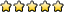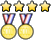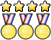## Astronomy C

AlphaTauri
Staff EmeritusPosts: 829
Joined: September 11th, 2009, 1:41 pm
State: PA
Location: 04h 35m 55.239s, +16° 30′ 33.49″
Has thanked: 0
Been thanked: 0
Contact:

### Re: Astronomy C

Unome wrote:
Magikarpmaster629 wrote:Nice test, AlphaTauri, but what are EGGs? I haven't been able to find what they are.
Is it something other than this?
Yes, that is indeed what they are.

Also, glad to hear that you liked the test, Magikarpmaster!
Hershey Science Olympiad 2009 - 2014
Volunteer for Michigan SO 2015 - 2018

]\/[ Go Blue!

Magikarpmaster629
Exalted MemberPosts: 578
Joined: October 7th, 2014, 3:03 pm
State: MA
Location: No idea, but I can tell you exactly how fast I'm going
Has thanked: 0
Been thanked: 1 time

### Re: Astronomy C

AlphaTauri wrote: Also, glad to hear that you liked the test, Magikarpmaster!
Yeah; my partner and I got a 55%. We missed a lot of the math stuff (never expected Jeans mass to show up) and the more obscure concept questions.

A wild goose flies over a pond, leaving behind a voice in the wind.
A man passes through this world, leaving behind a name.

ModeratorPosts: 493
Joined: December 6th, 2013, 1:56 pm
State: TX
Pronouns: He/Him/His
Location: Austin, Texas
Has thanked: 16 times
Been thanked: 16 times

### Re: Astronomy C

andrewwski wrote:
sciolymom wrote:Regarding high mass versus low mass stars and their affect on planets:

First question - one of the objects connected a short orbital period with the low mass of the host star. I'm not understanding how that is connect.

Second question - I always thought that higher mass stars were more likely to have tidally locked planets due to their significant gravity. But I read this elsewhere:
On the opposite extreme, stars with less than half of Sol's mass are more likely to tidally lock planets that are orbiting close enough to have liquid water on their surface too quickly, before life can develop (Peale, 1977).

I have a feeling these two questions might be related... I would think that if low mass stars tend to have planets closer to the host, the closeness is what causes the tidal locking. But what is it that makes them end up closer to the low mass star than to a high mass star?
For your first question - let's start by looking at Newton's Law of Universal Gravitation:

If is the mass of the star and is the mass of the planet, the force due to gravity can be determined if you know the distance between the two, .

Now, this doesn't necessarily say anything about an orbit. It applies whether or not the objects are in orbit. If we were to apply Newton's Second Law to this, we could derive the equation of motion for the two-body problem, and then determine analytically the conditions for which there is an elliptical orbit - but that would be beyond the scope of this event.

However, if you think about the notion of a circular orbit, you will realize that the gravitational force must balance with the centripetal force, which is . So for any given distance from the star , the gravitational force can be determined, and thus there is one velocity that corresponds to a circular orbit at this distance. This concept could be extended for an elliptical orbit, but let's think about the circular case as it's more straightforward to visualize.

Understanding this, if we were to take the pure two-body problem - i.e. we assume that the only two bodies undergoing gravitational attraction are those corresponding to and , then in theory we can have an orbit for any distance and any mass .

But, we never encounter the perfect two-body problem in the universe. There are always other "perturbing" forces - for example, gravity due to other objects, or force due to radiation pressure from the star, etc. These forces are in addition to the gravitational force to the central star.

If the gravitational force between the planet and star are high compared to the perturbing forces, then the perturbing forces will have a minimal effect. However, if the gravitational force is not high compared to the perturbing forces, then they will have a large effect. At some point, they will make it such that the object is no longer orbiting the central star.

So, for a low-mass star, this means that you expect to see objects orbiting closer to it than a high-mass star. Since is smaller, must be increased (or , or likewise , must be decreased) - so these planets will be closer to the star!

As you get closer to the star (the orbital radius shrinks), the orbital period decreases. Note that orbital period is given by:

where is the standard gravitational parameter .

is the semimajor axis, which is equal to the radius for a circular orbit - so thus as decreases, so does the period. This is intuitive - the velocity must be faster as the orbit becomes smaller.

Your second question follows from this. Let's consider why tidal locking happens - it is due to a gravity gradient within the planet. If the mass of the planet is not uniformly distributed, gravity will have a stronger pull on the part with a higher mass concentration. Since planets tend to not have perfectly symmetrical density, or be a perfect sphere, gravity will act more on one part of the planet than another.

But the question is - how much more? Let's look again at Newton's Law of Gravitation - we see that as M increases, F increases linearly, but as r increases, F increases to the power of two! So the distance from the star has more of an effect than the mass of the star!

Thus, the distance of the point from the center of mass of the central star (assuming M >> m) has the greatest effect on the gravitational force. As you get closer to the star, the effect of moving one unit closer to the sun in has a greater and greater effect on the gravitational force.

Let's look at the solar system, for example. If we look at Mercury, for example, we see that it is km from the sun, and has a radius of 2440 km. Then, let's look at the difference in gravitational force on the surface of Mercury closest to the sun:

or, if we divide these out, the gravitational force will be % greater at the closer surface than the far surface.

Now, let's take Jupiter, for example, which is much, much larger than Mercury but also much further from the sun. It is about km from the sun with a radius of 69,911 km. If we do the same, we find:

or the gravity at the surface of Jupiter closer to the sun is % greater than at the far surface of Jupiter. Compare this to Mercury, even though Jupiter has a radius over 28x larger than Mercury!

So, you can see that the closer a planet is to a star, the greater the gravity gradient, and thus the more likely it is to be tidally locked. As we discussed before, a lighter star is more likely to have planets orbiting closer to it.

Hope this makes sense!
HOLY CRAP HOW DID I MISS THIS thanks so much andrewwski that makes a ton of sense
Magikarpmaster629 wrote:
AlphaTauri wrote: Also, glad to hear that you liked the test, Magikarpmaster!
Yeah; my partner and I got a 55%. We missed a lot of the math stuff (never expected Jeans mass to show up) and the more obscure concept questions.
Took it, got a 68%. What an awesome (but hard T_T) test. Also yeah magikarpmaster629 it was super cool to have random concepts I had never heard of show up like Poynting Robertson drag. AlphaTauri, thanks for both writing and sharing this test! It was a pleasure to take.
University of Texas at Austin '22
Seven Lakes High School '18
Beckendorff Junior High '14

Magikarpmaster629
Exalted MemberPosts: 578
Joined: October 7th, 2014, 3:03 pm
State: MA
Location: No idea, but I can tell you exactly how fast I'm going
Has thanked: 0
Been thanked: 1 time

### Re: Astronomy C

Looking at radial velocity plots and this website: here eccentricity obviously affects the radial velocity of a star. However, I haven't been able to find an equation for eccentricity through a radial velocity curve. Is it calculable with just the curve?

Also, if I was asked to find the habitable zone around the star, I would just find the semi-major axes of planets with equilibrium temperatures at 273 and 373, right?

A wild goose flies over a pond, leaving behind a voice in the wind.
A man passes through this world, leaving behind a name.

doge
MemberPosts: 8
Joined: December 11th, 2013, 7:10 pm
Division: C
State: DC
Has thanked: 0
Been thanked: 0

### Re: Astronomy C

Magikarpmaster629 wrote:Looking at radial velocity plots and this website: here eccentricity obviously affects the radial velocity of a star. However, I haven't been able to find an equation for eccentricity through a radial velocity curve. Is it calculable with just the curve?
If you somehow had the radial velocities at apoastron and periastron, then you could find the ratio of the velocity at apoastron to the velocity at periastron to find the ratio (1-e)/(1+e) and solve for e that way. But this requires radial velocity graph to be determined at a very specific orientation of the system with the apoastron occurring at the max positive/negative radial velocity and the periastron occurring at the maximum of the other as other orientations would mess up the relative velocities for the entire orbit. Otherwise I don't know, maybe you could use some calculus to find it.
Magikarpmaster629 wrote:Also, if I was asked to find the habitable zone around the star, I would just find the semi-major axes of planets with equilibrium temperatures at 273 and 373, right?
I doubt a testmaker would give you a pressure other than 1 atm, so yes.

ModeratorPosts: 493
Joined: December 6th, 2013, 1:56 pm
State: TX
Pronouns: He/Him/His
Location: Austin, Texas
Has thanked: 16 times
Been thanked: 16 times

### Re: Astronomy C

doge wrote:
Magikarpmaster629 wrote:Also, if I was asked to find the habitable zone around the star, I would just find the semi-major axes of planets with equilibrium temperatures at 273 and 373, right?
I doubt a testmaker would give you a pressure other than 1 atm, so yes.
In the event that they do, you can find rough approximation of what temperature it would be using a phase diagram. This is a good one I used a lot in solar system last year:For example, if you have a lower atmospheric pressure (e.g. Denver has a pressure of around 0.85 atm iirc), water will boil at a lower temperature. On Mt. Everest, it's only about 71C, while at Denver it's about 95C. So if a planet doesn't have an atmosphere at all, it'll be impossible for water to exist in a stable configuration on the surface as a liquid, since it'd be a (near?) vacuum. Instead, it would be a gas or solid.

This is pretty cool because often when calculating equilibrium temperatures we assume stuff like no atmosphere/greenhouse effect. However, even if the equilibrium temperature without an atmosphere is in the right range, if there's no atmosphere to have pressure (which would likely make the planet hotter unless it's all white clouds?) there won't be any water.
University of Texas at Austin '22
Seven Lakes High School '18
Beckendorff Junior High '14

syo_astro
Exalted MemberPosts: 601
Joined: December 3rd, 2011, 9:45 pm
State: NY
Has thanked: 0
Been thanked: 5 times
Contact:

### Re: Astronomy C

So after some looking up stuff just to link a helpful source to respond to Karp's question about eccentricity and orbits: https://web.njit.edu/~gary/320/Lecture6.html (ignore some of the derivations as they are a bit tough and quite a bit of algebra/some calculus...but some of the key results at the bottom of the page might be useful to search up about)

As I recall there was also a question Cicc had one one of his States tests (I think the one from just last year) that involved conservation of energy in relation to your question.

I may post more if there's deeper questions about this, but the only other thing I can think of that might be of interest is that interestingly the eccentricity of two objects in a binary system (eg. a binary star...or in context of this year a star-planet system) is equal. You can think of this because the distance from the center of mass to an object is linearly proportional to the mass of a given object. This goes for both objects of the system, so inc mass -> inc. separation -> inc eccentricity equivalently of both orbits (one can also think of this in context of Kepler's Laws to understand about what other quantities are equivalent for a system, namely period). At least this should be true for simpler problems (I'm sure life can always make things more complex as doge suggested), so hopefully this also provides something of interest to think about/search up!
B: Crave the Wave, Environmental Chemistry, Robo-Cross, Meteorology, Physical Science Lab, Solar System, DyPlan (E and V), Shock Value
C: Microbe Mission, DyPlan (Earth's Fresh Waters), Fermi Questions, GeoMaps, Gravity Vehicle, Scrambler, Rocks, Astronomy

Unome
ModeratorPosts: 4253
Joined: January 26th, 2014, 12:48 pm
State: GA
Location: somewhere in the sciolyverse
Has thanked: 85 times
Been thanked: 32 times

### Re: Astronomy C

So on our state test we had a question giving us apparent magnitude and distance from earth of an object (they were 7.36 and 90 parsecs if I remember correctly), and were asked for luminosity. We ended up pulling a good-looking equation out of our binders and checking it against the next problem (which gave us two typical values for different luminosity classes and asked us to identify the class), but I can't figure out how to do that problem (nor do I understand how luminosity even works, so...)
Userpage
Chattahoochee High School Class of 2018
Georgia Tech Class of 2022

Opinions expressed on this site are not official; the only place for official rules changes and FAQs is soinc.org.

Magikarpmaster629
Exalted MemberPosts: 578
Joined: October 7th, 2014, 3:03 pm
State: MA
Location: No idea, but I can tell you exactly how fast I'm going
Has thanked: 0
Been thanked: 1 time

### Re: Astronomy C

Luminosity is the amount of energy radiated by a star (or any object really) per second, or power in watts. This value is determined by the Stephan-Boltzmann law; based on the radius and surface temperature of the star.

Absolute magnitude is a logarithmic scale of luminosity; it is used to determine how many times brighter a star is than another one. This can be calculated with the equation: where L(sun) is the luminosity of the star in solar luminosities (divide the number of watts of the star by the watts the sun emits) and M is the absolute magnitude. It can easily be determined that lower values refer to more luminosity, and this scale goes into negative numbers.

Apparent magnitude is a similar scale to absolute magnitude, but it is based on how bright a star appears, not how bright it actually is. Since light follows the inverse square law, objects more distant will appear less bright than up close. In order to have a standard, the absolute magnitude of a star is its apparent magnitude at a distance of ten parsecs. The apparent magnitude scale is similar to absolute magnitude, calculated with where m is the apparent magnitude and I is the incident flux received from the star. This means that five steps in apparent magnitude refers to appearing 100 times as bright. The distance modulus relates the apparent and absolute magnitudes with the distance to the system: where m is the apparent magnitude, M is the absolute magnitude and D is the distance to the system in parsecs.

Now let's look at your problem. Using the distance modulus and flipping around the variables, we get an absolute magnitude of 2.59. Solving for luminosity in the absolute magnitude-luminosity equation, we get 7.46 solar luminosities. If the question asks for the answer in watts, just multiply by the solar luminosity.

A wild goose flies over a pond, leaving behind a voice in the wind.
A man passes through this world, leaving behind a name.

Unome
ModeratorPosts: 4253
Joined: January 26th, 2014, 12:48 pm
State: GA
Location: somewhere in the sciolyverse
Has thanked: 85 times
Been thanked: 32 times

### Re: Astronomy C

Magikarpmaster629 wrote:Luminosity is the amount of energy radiated by a star (or any object really) per second, or power in watts.
Whoa really? I seriously never knew this. Looking at your answer, I think we got it correct (-ish), so yay!
Userpage
Chattahoochee High School Class of 2018
Georgia Tech Class of 2022

Opinions expressed on this site are not official; the only place for official rules changes and FAQs is soinc.org.

### Who is online

Users browsing this forum: No registered users and 1 guest